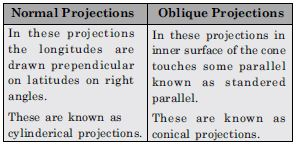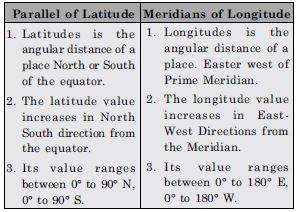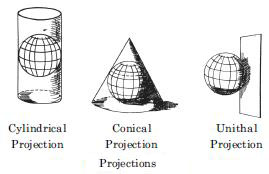# NCERT Solutions for Class 11 Geography Chapter 17 - Map Projections

##### Question 1:

A map projection least suitable for the world map:

1. Mercator
2. Simple Cylindrical
3. Conical
4. All the above

(c). Conical

##### Question 2:

A map projection that is neither the equal area nor the correct shape and even the directions are also incorrect.

1. Simple Conical
2. Polar zenithal
3. Mercator
4. Cylindrical

(d). Cylindrical

##### Question 3:

A map projection having correct direction and correct shape but area greatly exaggerated polewards is

1. Cylindrical Equal Area
2. Mercator
3. Conical
4. All the above

(b). Mercator

##### Question 4:

When the source of light is placed at the centre of the globe, the resultant projection is called.

1. Orthographic
2. Stereographic
3. Gnomonic
4. All the above

(c). Gnomonic

##### Question 5:

Describe the elements of map projection.

The elements of map projection are as following:
1. Reduced earth: The earth’s model is represented with the help of a reduced scale on a flat sheet of paper on this model the network of graticule can be translated.
2. Parallels of latitude: These are the circles round the globe parallel to the equator and maintaining uniform distance from the poles. They are demarcated as 0° to 90° North and South.
3. Meridians of longitudes: Longitues are the elements of map projections. There are also drawn north to south direction from one pole to another and two opposite meridians make a complete circle.
4. Global property: Distance between any given points of a region shape of the region, size or area of the region in accuracy are given in it.

##### Question 6:

What do you mean by global property?

The global property means the
1. Correctners of the area
2. Direction
3. Distance
4. and shape of the area.

##### Question 7:

Not a single map projection represents the globe truly. Why?

Map projection project the network of latitudes and longitudes on a plain surface by using the geometrical and mathematical methods. The globe is the true representation of earth. The projections given a shadowed picture of the globe which is distorted in those parts which are further away from the point where the paper touches the globe.

##### Question 8:

How is the area kept equal in cylindrical equal area projection?

The area is kept equal in cylindrical equal area projection because the parallels and meridians are projected as straight lines intersecting one another at right angles.

##### Question 9:

Differentiate between: Developed and non-developed surface.

Developed Surface: A developed surface is a surface which can be unrolled into a plane surface. For e.g. a plane, a cylinder and a cone are developable surfaces.
Undeveloped surface: An undeveloped surface can not unroll into a place just as a globe.

##### Question 10:

Differentiate between: Homolographic and Orthographic projections.

Homolographic projection: These are the projections which are graticule of latitudes and longitudes on the map its equal in area. Orthographic Projection: On these projections the correct shape of the area of a country is shown.

##### Question 11:

Differentiate between: Normal and Oblique projections.##### Question 12:

Differentiate between: Parallels of latitudes and meridians of longitues.##### Question 13:

Discuss the criteria used for classifying map projection and state the major characteristics of each type of projection.

Map Projections: Globe is the best and accurate model of the earth. There are many problems in representing the spherical globe on a flat sheet of paper. Map projections are the means of transferring the lines of latitude and lines of longitude of the globe on to a flat sheet of paper. The expansion of a photograph on a screen in a cinema hall is an example of Projection. By this method, the lines of latitude and lines of longitude are projected on a flat sheet of paper. Classification of map projections: Different types of map projections are used. These are classified on the following bases:
1. On the basis of source of light: On this basis, the projections are of two types:
(a) Perspective projections
(b) Non-Perspective projections.
Perspective projections are prepared on the basis of Geometry. In these projections, shadow of lines of latitude and longitude are drawn on the basis of the location of source of light. Source of light can be placed at different positions.
Non-perspective projections are called mathematical projections. In these projections, source of light is not used. Principles of mathematics are used to draw these projections.
2. On the basis of purpose:
(a) Equal-area Projection: With the help of these projections, the equal-area property of a map is preserved. These are called Homolographic projections also. These are used for preparing distribution maps.
(b) Orthomorphic Projections: On these projections, the correct shape of an area or a country is shown.
(c) Azimuthal Projections: These map projections help to preserve the property of correct direction on a map. These are used for preparing maps for sailors and for air-navigators.
3. On the basis of construction:
(a) Cylindrical Projections: In these projections, it is imagined that a hollow cylinder circumscribes the globe. The equator of the globe touches the cylinder so that the length of the equator remains correct. In this group, the following non-perspective projections are included:
(i) Simple cylindrical projection
(ii) Cylindrical equal area projection
(iii) Mercator’s projection.In these projections, lines of latitude are parallel to the equator and are straight lines. The meridians are vertical straight-lines. These lines intersect each other at right angles. (b) Conical Projections: In this projection, the cone is used as a surface. It is imagined that the cone is placed on the globe so that its vertex is above the pole. The axis of the cone coincides with that of the globe. The parallel along which the cone touches the globe is called the standard parallel. It keeps correct length. The parallels are shown by concentric arcs of circles. Meridians are straight lines drawn at equal angular distance.
In this group, the following map projections are included:
(i) Simple Conical Projection with one standard parallel.
(ii) Conical Projection with two standard parallels
(iii) Bonne’s Projection
(iv) Polyconic Projection.
(c) Zenithal Projections: In these projections, it is assumed that a plane surface is placed tangentially at the pole of the globe. These are azimuthal projections showing correct bearing. These are used for polar areas. According to the position of the source of light, these projections are perspective projections.
(i) Polar Zenithal Gnomonic Projection.
(ii) Polar Zenithal stereographic Projection.
(iii) Polar Zenithal orthographic Projection.
In this group, two non-perspective zenithal projections of zenithal equidistant projection and zenithal equal area projection are also included.
(d) Conventional Projection: These projections are mathematical projections. These are called conventional projections, because no surface is used in these. These are drawn for specific purpose of drawing world maps. These include the Sinusoidal projection and Mollweide’s projections.
Importance of Map Projections
(i) Map projections are useful for drawing maps on a flat sheet of paper.
(ii) These provide knowledge about the true shape of the earth.
(iii) Atlas maps and wall maps are prepared on the basis of map projections.
(iv) Different types of maps are prepared with the help of map projections.
(v) These help us to know the location of lines of latitude and lines of longitude.
(vi) Map projections are used to draw distribution maps, political maps, and maps of ocean routes, air routes and ocean currents.

##### Question 14:

Which map projection is very useful for navigational pruposers? Explain the main properties and limitations of this projection?

The Mercater projection is an orthomorphic projection and very useful for the navigational purposes. The properties and limitations are as under:
Properties of Mercator’s Projection
(1) It is a cylindrical projection which was developed by a Dutch Cartographer Mercator in 1569.
(2) It is a non-perspective projection.
(3) The parallels are straight lines parallel and equal to Equator.
(4) Meridians are perpendiculars drawn at equal distances.
(5) Meridians and parallels intersect at right-angles.
(6) Scale along equator is correct. But it increases along other parallels as we move towards poles.
Pole is a point but it is drawn equal to equator. So, it is exaggerated infinite.
(7) Scale along meridians is also exaggerated.
Limitations:
(1) Poles can not be shown on this projection.
(2) The scale in polar area is exaggerated many times.
So the area is increased in polar areas.

##### Question 15:

Discuss the main properties of conical projection with one standard parallel and discribe its major limitations.